# Precalculus : Evaluate Expressions That Include the Inverse Sine or Cosine Function

## Example Questions

### Example Question #1 : Evaluate Expressions That Include The Inverse Sine Or Cosine Function

Find angle A of the following triangle: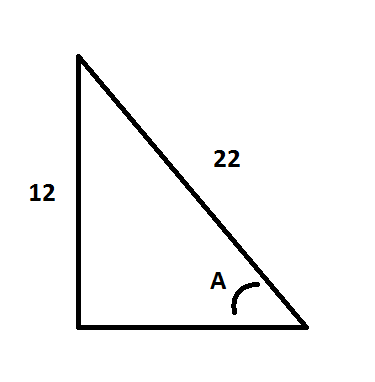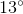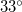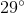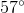Explanation:

We are given the hypotenuse and the side opposite of the angle in question. The trig function that relates these two sides is SIN. Therefore, we can write: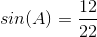In order to solve for A, we need to take the inverse sin of both sides: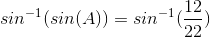which becomes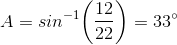### Example Question #2 : Inverse Sine And Cosine Functions

Consider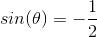, where theta is valid from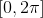.  What is a possible value of theta?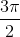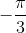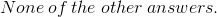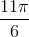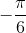Explanation:

Solve for theta by taking the inverse sine of both sides.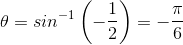Since this angle is not valid for the given interval of theta, addradians to this angle to get a valid answer in the interval.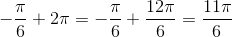### Example Question #1 : Evaluate Expressions That Include The Inverse Sine Or Cosine Function

Evaluate: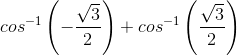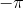Explanation:

First evaluate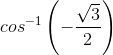.

To evaluate inverse cosine, it is necessary to know the domain and range of inverse cosine.

For: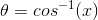The domainis only valid from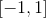.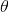is only valid from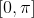.

The part is asking for the angle where the x-value of the coordinate is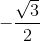.  The only possibility on the unit circle is the second quadrant.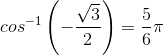Next, evaluate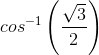.

Using the same domain and range restrictions, the only valid angle for the given x-value is in the first quadrant on the unit circle.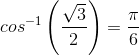Therefore: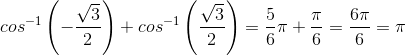### Example Question #1 : Evaluate Expressions That Include The Inverse Sine Or Cosine Function

Evaluate: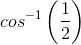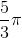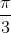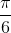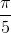Explanation:

To find the correct value of, it is necessary to know the domain and range of inverse cosine.

Domain:Range:The question is asking for the specific angle when the x-coordinate is half.

The only possibility is located in the first quadrant, and the point of the special angle is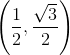The special angle for this coordinate is.

### Example Question #1 : Inverse Sine And Cosine Functions

Find the value of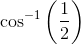.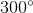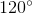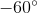Explanation:

In order to determine the value or values of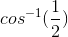, it is necessary to know the domain and range of the inverse sine function.

Domain:Range:The question is asking for the angle value of theta where the x-value isunder the range restriction.  Since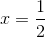is located in the first and fourth quadrants, the range restriction makes theta only allowable from.  Therefore, the theta value must only be in the first quadrant.

The value of the angle when the x-value isisdegrees.

### Example Question #3 : Evaluate Expressions That Include The Inverse Sine Or Cosine Function

Find the inverse of the function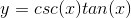Make sure the final notation is only in the forms including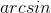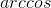, and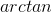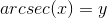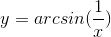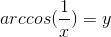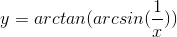Explanation:

The easiest way to solve this problem is to simplify the original expression.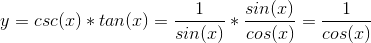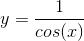To find its inverse, let's exchangeand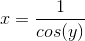Solving for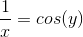### All Precalculus Resources